# The simplest rotation

To motivate many of our later developments, we start with the simplest case of a rotation: a rotation about a fixed axisthrough an angle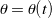. This example should be familiar to you from many different venues. To describe this rotation, we consider the action of this rotation on the set of fixed, orthonormal, right-handed basis vectors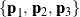. As depicted in Figure 1, we suppose that these vectors are transformed to the set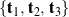by the rotation.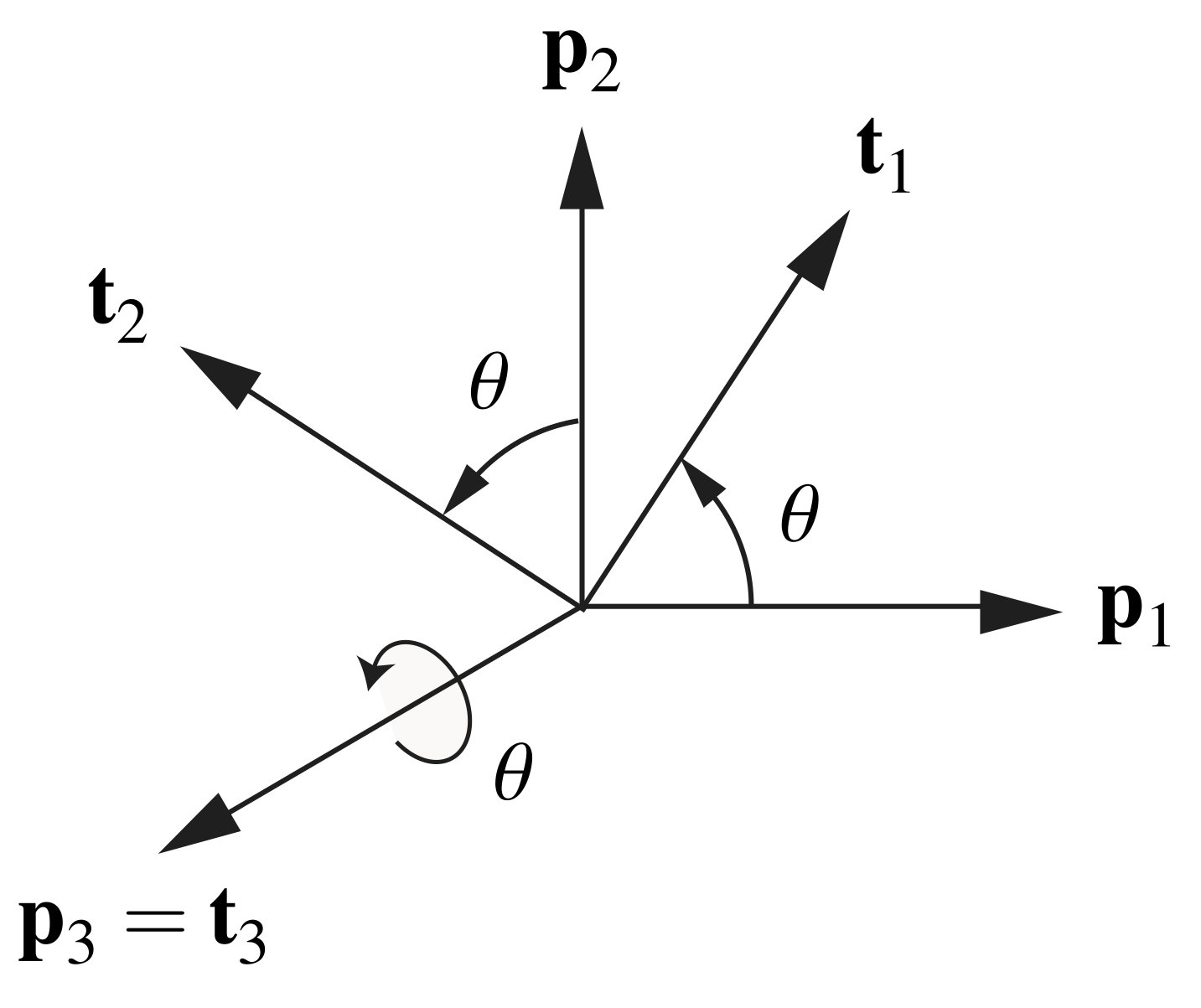Figure 1. The transformation of basis vectors into by a rotation about through an angle .

## A matrix representation of the rotation

Using a matrix notation, we can represent the transformation from the set of basis vectorstoas

(1)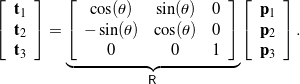It is easy to see that the matrix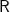in (1) has a unit determinant, and its inverse is its transpose: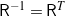. That is, the matrixis proper-orthogonal. By differentiating (1) with respect to time, we find that

(2)Using the fact that, we can easily replace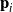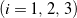in (2) with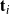to obtain

(3)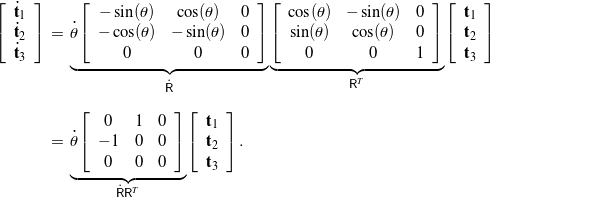Notice that this is equivalent to the familiar results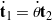and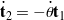from cylindrical polar coordinates. It should also be clear from (3) that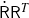is a skew-symmetric matrix. A vector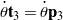can be introduced that has the useful property that

(4)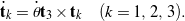You should notice how the vectorcan be inferred from the components of.

## A tensor representation of the rotation

It is convenient to use a tensor notation1 to describe the rotation we have been discussing. In particular, we can write (1) in the form

(5)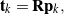where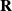is the tensor

(6)It can be shown that this representation of the rotation is equivalent to (1). Furthermore, because

(7)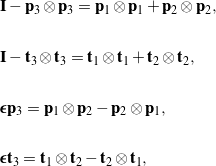we can express the tensorentirely in terms of the axis of rotation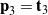and the angle of rotation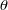:

(8)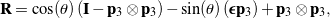or, equivalently,

(9)As we shall see later, these equivalent representations naturally lead to the general form of a tensor that represents a rotation about an arbitrary axis through an arbitrary angle of rotation. As with the matrix representation of this simple rotation, the rotation tensoris a proper-orthogonal tensor because it has a determinant of one and its inverse is its transpose: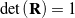and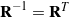. Differentiating (5), we find that

(10)where we have used the fact that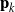are constant vectors. Some straightforward computations involving (6) reveal that

(11)and

(12)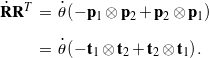With the help of these results, we conclude from (10) that

(13)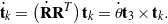As expected, this result is in agreement with (4). Expression (13) implies that the vectoris the axial vector of the skew-symmetric tensor.